# Grade - math word problems

#### Number of examples found: 5337

• Integers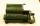May be the sum of two integers less than their difference?
• Logarithmic equation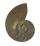Solve equation: log33(3x + 21) = 0
• 15 numberWhat number is smaller (greater) by 15 than its half?
• Angles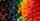Which of those angles are obtuse?
• Valid numberRound the 453874528 on 2 significant numbers.
• Tenths digit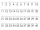For 10.932, which digit is in the tenths place?
• AnnulusThe radius of the larger circle is 8cm, the radius of smaller is 5cm. Calculate the contents of the annulus.
• EquationSolve the equation: 1/2-2/8 = 1/10; Write the result as a decimal number.
• If-then equationIf 5x - 17 = -x + 7, then x =
• The determinant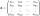The determinant of the unit matrix equals 7. Check how many rows the A matrix contains.
• EquationEquation ? has one root x1 = 8. Determine the coefficient b and the second root x2.
• Strange x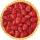For what x is true ??
• Write 2Write 791 thousandths as fraction in expanded form.
• Flour2 kg of flour costs 100 CZK. How much does it cost half a kilogram?
• Linear systemSolve this linear system (two linear equations with two unknowns): x+y =36 19x+22y=720
• Rotating coneCalculate volume of a rotating cone with base radius r=12 cm and height h=7 cm.
• 1.5 divided1.5 divided by 1 = w divided by 4
• Substitutionsolve equations by substitution: x+y= 11 y=5x-25
• Unknown number 23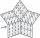Find 2/3 of unknown number, which is two thirds of the 99.
• Fit ballWhat is the size of the surface of Gymball (FIT - ball) with a diameter of 65 cm?

Do you have an interesting mathematical word problem that you can't solve it? Submit a math problem, and we can try to solve it.

We will send a solution to your e-mail address. Solved examples are also published here. Please enter the e-mail correctly and check whether you don't have a full mailbox.

Please do not submit problems from current active competitions such as Mathematical Olympiad, correspondence seminars etc...